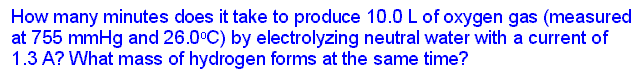$$\newcommand{\vecs}{\overset { \rightharpoonup} {\mathbf{#1}} }$$ $$\newcommand{\vecd}{\overset{-\!-\!\rightharpoonup}{\vphantom{a}\smash {#1}}}$$$$\newcommand{\id}{\mathrm{id}}$$ $$\newcommand{\Span}{\mathrm{span}}$$ $$\newcommand{\kernel}{\mathrm{null}\,}$$ $$\newcommand{\range}{\mathrm{range}\,}$$ $$\newcommand{\RealPart}{\mathrm{Re}}$$ $$\newcommand{\ImaginaryPart}{\mathrm{Im}}$$ $$\newcommand{\Argument}{\mathrm{Arg}}$$ $$\newcommand{\norm}{\| #1 \|}$$ $$\newcommand{\inner}{\langle #1, #2 \rangle}$$ $$\newcommand{\Span}{\mathrm{span}}$$ $$\newcommand{\id}{\mathrm{id}}$$ $$\newcommand{\Span}{\mathrm{span}}$$ $$\newcommand{\kernel}{\mathrm{null}\,}$$ $$\newcommand{\range}{\mathrm{range}\,}$$ $$\newcommand{\RealPart}{\mathrm{Re}}$$ $$\newcommand{\ImaginaryPart}{\mathrm{Im}}$$ $$\newcommand{\Argument}{\mathrm{Arg}}$$ $$\newcommand{\norm}{\| #1 \|}$$ $$\newcommand{\inner}{\langle #1, #2 \rangle}$$ $$\newcommand{\Span}{\mathrm{span}}$$$$\newcommand{\AA}{\unicode[.8,0]{x212B}}$$

In every electrochemical process, whether spontaneous or not, a certain amount of electric charge is transferred during the oxidation and reduction. The half-reactions we have written for electrode processes include the electrons which carry that charge. It is possible to measure the rate at which the charge is transferred with a device called an ammeter.

An ammeter measures the current flowing through a circuit. The units of current are amperes (A) (amps, for short). Unlike a voltmeter, ammeters allow electrons to pass and essentially "clock" them as they go by. The amount of electric charge which has passed through the circuit can then be calculated by a simple relationship:

Charge = current x time OR Coulombs = amps x seconds

This enables us to connect reaction stoichiometry to electrical measurements. The principles underlying these relationships were worked out in the first half of the 19th century by the English scientist, Michael Faraday.The diagram shows how voltage and current might be measured for a typical galvanic cell but the arrangment is the same for any electrochemical cell. Notice that the voltmeter is placed across the electron conduit (i.e., the wire) while the ammeter is part of that conduit. A good quality voltmeter can be used in this way even though it might appear to be "shorting out" the circuit. Since electrons cannot pass through the voltmeter, they simply continue along the wire.

Both the voltmeter and ammeter are polarized. They have negative and positive terminals marked on them. Electrons are "expected" only in one direction. This is important in measurements of direct current (DC) such as comes out of (or goes into) electrochemical cells.

Faraday's law of electrolysis might be stated this way: the amount of substance produced at each electrode is directly proportional to the quantity of charge flowing through the cell. Of course, this is somewhat of a simplification. Substances with different oxidation/reduction changes in terms of the electrons/atom or ion will not be produced in the same molar amounts. But when those additional ratios are factored in, the law is correct in all cases.

Example 1Example 2Example 3Example 4Example 5